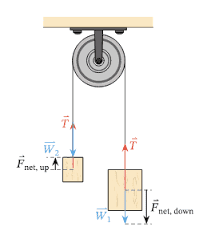# Tug of war applications using Newton's third law

• bbgur
In summary, Newton's third law does not always apply - in a tug of war with a hanging mass, if one person is winning, the tension in the rope is not the same in both directions.f

#### bbgur

Homework Statement
Will the action and reaction force are same in magnitude (sizes) when during tug of war if one person is winning? Will the force exterted by guy A opposite in direction to that exerted by guy B?
I understand the action and reaction force will be same if neither of them wins. But I am not sure when one person wins.
Relevant Equations
F = -F
F = -FWhy wouldn't Newton's third law apply? Even if someone wins?Why wouldn't Newton's third law apply? Even if someoneWhy wouldn't Newton's third law apply? Even if someone wins?
For scenario 1: if no one is winning based on Newton third law - the force will be same because every action has equal and opposite force.
Buy I am not too sure what will happen when one person is winning

Buy I am not too sure what will happen when one person is winning
Perhaps draw a free-body diagram for the scenario.

Perhaps draw a free-body diagram for the scenario.
Hi,
I understand that the frictional force determine the winner but does this mean that the action/ reaction force is not equal in magnitude (sizes)?

Hi,
I understand that the frictional force determine the winner but does this mean that the action/ reaction force is not equal in magnitude (sizes)?
Draw a FDB. Newton's third law applies. If you pull an object, the objects pulls you with the same force. That's why we have conservation of momentum (in a closed system).

Hint: think about a tug of war in space, with no ground to stand on. What would happen?

•Steve4Physics and bbgur
Homework Statement:: Will the action and reaction force are same in magnitude (sizes) when during tug of war if one person is winning?
I'd like to chip in.

There are 4 forces acting on contestant A (though 2 are unimportant here).

There are another 4 forces acting on contestant B (though 2 are unimportant here).

Do you know what the 8 forces are? The 'action and reaction' between A and B are 2 of these 8 forces.

Newton's 3rd law is always correct - but must, of course, be applied to the correct pair of forces.

Think about an extreme case: A is on rough concrete and B is on wet ice. Your free-body diagrams (for A and B) - with arrow-lengths showing the relative sizes of the different forces - should enable you to understand what's happening.

By the way, you shouldn't write "F = -F" (unless F happens to be zero).

•jbriggs444
Another way to look at it is this. Consider the two contestants together as the system. When one contestant starts "winning" this means that the two-contestant system starts accelerating in the direction of the net force acting on it. Where does this net force come from? Hint: Something external to the two-contestant system.

...
I understand the action and reaction force will be same if neither of them wins. But I am not sure when one person wins.

Replace persons with hanging masses.
The magnitude of the tension inside the rope must be the same in both directions (third law).
Since the total mass of the system abandons its initial state of repose, and starts moving in the direction of the bigger mass, the net force acting on the system of masses and rope can’t be zero (second law).if one person is winning? Will the force exterted by guy A opposite in direction to that exerted by guy B?
The only forces the contestants exert directly on each other are a tiny mutual gravitational attraction, and that will be equal and opposite on them.
A exerts a force FA on the rope and, by Newton III, the rope exerts a force -FA on A. Similarly forces FB, -FB between B and the rope.

When the system is not accelerating, the net force on the rope is zero, so FA+FB=0.
If we take the rope to be of negligible mass then, again, FA+FB=0, even if the system is accelerating.

It would be a mistake to ignore the rope and apply Newton III to the contestants directly since they are not in contact.